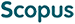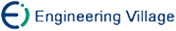张 忠 李传东. 具有时变时滞的递归神经网络的渐近稳定性分析[J]. 计算机研究与发展, 2007, 44(6): 973-979.
 引用本文: 张 忠 李传东. 具有时变时滞的递归神经网络的渐近稳定性分析[J]. 计算机研究与发展, 2007, 44(6): 973-979.Zhang Zhong and Li Chuandong. Asymptotical Stability Analysis for Recurrent Neural Networks with Time-Varying Delays[J]. Journal of Computer Research and Development, 2007, 44(6): 973-979.
 Citation: Zhang Zhong and Li Chuandong. Asymptotical Stability Analysis for Recurrent Neural Networks with Time-Varying Delays[J]. Journal of Computer Research and Development, 2007, 44(6): 973-979.## Asymptotical Stability Analysis for Recurrent Neural Networks with Time-Varying Delays

• 摘要: 当神经网络应用于最优化计算时，理想的情形是只有一个全局渐近稳定的平衡点，并且以指数速度趋近于平衡点，从而减少神经网络所需计算时间.研究了带时变时滞的递归神经网络的全局渐近稳定性.首先将要研究的模型转化为描述系统模型，然后利用Lyapunov-Krasovskii稳定性定理、线性矩阵不等式(LMI)技术、S过程和代数不等式方法，得到了确保时变时滞递归神经网络渐近稳定性的新的充分条件，并将它应用于常时滞神经网络和时滞细胞神经网络模型，分别得到了相应的全局渐近稳定性条件.理论分析和数值模拟显示，所得结果为时滞递归神经网络提供了新的稳定性判定准则.

Abstract: When the neural network applies to optimal calculation, the ideal situation is that there is a unique equilibrium point which is globally asymptotically stable and the neural network tends to the equilibrium point. The problem of the globally asymptotical stability of recurrent neural networks with time varying delay is investigated. By transforming the delayed neural model to the describer model and then employing the Lyapunov-Krasovskii stability theorem, linear matrix inequality (LMI) technique, S procedure, and some algebraic inequality method, a new sufficient condition is derived, which is determined by the coefficients of the model and includes more tuning parameters for determining the globally asymptotical stability of recurrent neural networks with time-varying delay. The condition is easily verified numerically by the interior-point algorithm for convex quadratic programming because it can be changed as a set of linear matrix inequalities. The proposed result is further applied to two special cases: cellular neural network model with time delay and recurrent neural networks with constant delays. It is shown by theoretical analysis and computer simulations that the presented results provide several new sufficient conditions for the asymptotical stability of the investigated delayed neural network model./下载:  全尺寸图片 幻灯片
• 分享
• 用微信扫码二维码

分享至好友和朋友圈